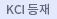#검색
다국어 입력

http://chineseinput.net/에서 pinyin(병음)방식으로 중국어를 변환할 수 있습니다.

변환된 중국어를 복사하여 사용하시면 됩니다.

예시)
• 中文 을 입력하시려면 zhongwen을 입력하시고 space를누르시면됩니다.
• 北京 을 입력하시려면 beijing을 입력하시고 space를 누르시면 됩니다.
닫기

검색결과 좁혀 보기

• 좁혀본 항목 보기순서

• 원문유무
• 음성지원유무
• 원문제공처
• 등재정보
• 학술지명
• 주제분류
• 발행연도
• 작성언어
• 저자

오늘 본 자료

• 오늘 본 자료가 없습니다.
더보기
• 무료
• 기관 내 무료
• 유료
•••지역별 특성에 따른 국민지적자본 측정지표 개발 : 광역시를 중심으로

'스콜라' 이용 시 소속기관이 구독 중이 아닌 경우, 오후 4시부터 익일 오전 7시까지 원문보기가 가능합니다.

• 추진축 체결용 유압식 커플링 볼트의 국산화

본 논문은 기존 Reamer Bolt 사용시 발생하는 여러가지 문제점을 해결하고 고가의 수입품을 대체하기 위한 유압식 커플링 볼트의 국산화를 소개하였다.

•논문 : 부식환경 ; 알칼리 수용액에서 Zr 합금의 산화막 성장기구

The autoclave corrosion test was performed on the zirconium alloys in various aqueous solutions of LiOH, NaOH, KOH, RbOH, and CsOH, The oxide growth mechanism at metal-oxide interface was evaluated using SEM on the samples which were prepared to have an equal oxide thickness at pre-transition regime and post transition regime. At a low concentration (4.3 mmol), the corrosion rate was gradually decreased as the ionic radius of cation increased. However. at a high concentration (32.5 mmol). the corrosion rate was significantly accelerated in LiOH solution and slightly in NaOH solution. The morphologies and the growth rates of the oxides at metal-oxide interface were dependent on the ionic radius of cation even if the samples have an equal oxide thickness in LiOH, NaOH, and KOH, The grain orientation in Zr matrix also controlled the growth rates and the morphologies of the oxides. Therefore. It is considered that the oxide growth mechanism of Zr alloys can be controlled by the cation incorporation into zirconium oxide.

•기계적 합금화법을 이용한 전이금속 도핑에 따른 TiO<sub>2</sub>분말의 광촉매 특성

Transition metal ions($Ni^{2+}$, $Cr^{3+}$ and $V^{5+}$) doped $TiO_2$ nanostructured powders were synthesized by mechanical alloying(MA) to shift the adsorption threshold into the visible light region. The synthesized powders were characterized by XRD, SEM, TEM and BET for structural analysis, UV-Vis and photoluminescence spectrum for the optical study. Also, photocatalytic abilities were evaluated by decomposition of 4-chlorophenol(4CP) under ultraviolet and visible light irradiations. Optical studies showed that the absorption wavelength of transition metal ions doped $TiO_2$ powders moved to visible light range, which was believed to be induced by the energy level change due to the doping. Among the prepared $TiO_2$ powders, $NiO^{2+}$ doped $TiO_2$ powders, showed excellent photooxidative ability in 4CP decomposition.

•균일침전시 여러 가지 금속염화물들을 첨가하여 제조된 TiO<sub>2</sub> 나노 분말들의 광산화 능력 평가

황두선,이남희,이희균,김선재,Hwang,D.,S,Lee,N.,H,Lee,H.,G,Kim,S.,J 한국재료학회 2004 한국재료학회지 Vol.14 No.4

Transition metal ions doped $TiO_2$ nanostructured powders were prepared with simply heating aqueous $TiOCl_2$ solutions, contained various metal ions (Ni, Al, Fe, Zr, and Nb) of 1.47 mol% added as metal-chlorides, at $100^{\circ}C$ for 4 hrs by homogeneous precipitation process under suppressing conditions of water vaporization. The characterizations for prepared $TiO_2$ powders were carried out to observe doping of metal ions, their concentrations and microstructures using XRD, UV-VIS (DRS), XPS, SEM, TEM and ICP. Also, photo-oxidative abilities were evaluated by decomposition of 4-chlorophenol (4CP) under ultraviolet light irradiations. No secondary oxide phases were formed in all the $VTiO_2$ powders, showing doping with various transition metal ions. When adding ions ($Ni^{2+}$ or$Al^{3+ }$ and $Zr^{4+}$ ) having valance states or ionic radii greatly different from those of $Ti^{4+}$ , the $TiO_2$ powders of mixed anatase and rutile phases were formed, whereas in the case of additions of $^Fe{3+ }$ and $Nb^{ 5+}$ as well as no addition of metal ion the powders with pure rutile phase alone were formed. Among the prepared $TiO_2$ powders, Ni$^{2+}$ doped $TiO_2$ powders, containing a small amount of anatase phase, showed excellent photo-oxidative ability in 4CP decomposition because of relative decreases in electron-hole recombination and poisoning of $TiO_2$ surface during the photoreaction.n.

•전이금속원소들이 첨가된 나노 티타니아 졸 및 코팅막 제조

이강,이남희,신승한,이희균,김선재,Lee,K.,Lee,N.,H.,Shin,S.,H.,Lee,H.,G.,Kim,S.,J. 한국재료학회 2004 한국재료학회지 Vol.14 No.9

The photocatalytic performance of $TiO_2$ thin films coated on porous alumina balls using various aqueous $TiOCl_2$ solutions as starting precursors, to which 1.0 $mol\%$ transition metal ($Ni^{2+},\;Cr^{3+},\;Fe^{3+},\;Nb^{3+},\;and\;V^{5+}$) chlorides had been already added, has been investigated, together with characterizations for $TiO_2$ sols synthesized simultaneously in the same autoclave through hydrothermal method. The synthesized $TiO_2$ sols were all formed with an anatase phase, and their particle size was between several nm and 30 nm showing ${\zeta}-potential$ of $-25{\sim}-35$ mV, being maintained stable for over 6 months. However, the $TiO_2$ sol added with Cr had a much lower value of -potential and larger particle sizes. The coated $TiO_2$ thin films had almost the same shape and size as those of the sol. The pure $TiO_2$ sol showed the highest optical absorption in the ultraviolet light region, and other $TiO_2$ sols containing $Cr^{3+},\;Fe^{3+}\;and\;Ni^{2+}$ showed higher optical absorption than pure sol in the visible light region. According to the experiments for removal of a gas-phase benzene, the pure $TiO_2$ film showed the highest photo dissociation rate in the ultraviolet light region, but in artificial sunlight the photo dissociation rate of $TiO_2$ coated films containing $Cr^{3+},\;Fe^{3+}\;and\;Ni^{2+}$ was measured higher together with the increase of optical absorption by doping.